Article Index

4. Examples

These examples are a continuation of those from the Latitudes and Departures chapter.

a. Traverse with BearingsFigure E-6 Bearing Traverse Example

 Line Bearing Length (ft) Lat (ft) Dep (ft) AB S 68°05'35"W 472.68 -176.357 -438.548 BC N 19°46'00"W 216.13 +203.395 -73.093 CD N 45°55'20"E 276.52 +192.357 +198.651 DA S 54°59'15"E 382.24 -219.312 +313.065 sums: 1347.57 +0.083 +0.075 Distance Lat err too far N Dep err too far E

(1) Adjust the Lats and Deps

Setup Equations E-1 and E-2: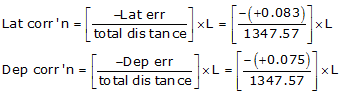Now solve Equations E-3 and E-4 for each line:

Line AB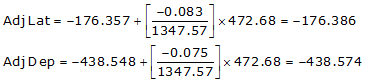Line BC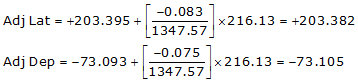Line CD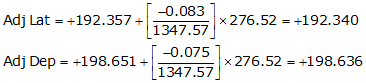Line DACheck the closure condition

 Adjusted Line Lat (ft) Dep (ft) AB -176.386 -438.574 BC +203.382 -73.105 CD +192.340 +198.635 DE -219.336 +313.044 sums: 0.000 0.000 check check

A common mistake is to forget to negate Lat err and Dep err in the correction equations. If that happens, the closure condition will be twice what it originally was as the corrections were applied in the wrong direction.

(2) Compute adjusted lengths and directions

Use Equations E-5 and E-6 along with Figure E-5 to compute the new length and direction for each line.

Line AB

Adj Lat = -176.386 <- South
Adj Dep = -438.574 <- WestBecause it's the SW quadrant, Brng =S 68°05'27.4" W.

Line BC

Adj Lat = +203.382 <- North
Adj Dep = -73.105 <- WestBecause it's the NW quadrant, Brng = N 19°46'14.9" W

Line CD

Adj Lat = +192.340 <- North
Adj Dep = +198.635 <- East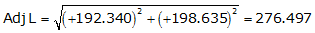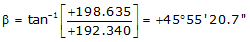Because it's the NE quadrant, Brng = N 45°55'20.7" E

Line DA

Adj Lat = -219.336 <- South
Adj Dep = +313.044 <- East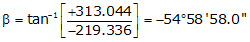Because it's the SE quadrant, Brng = S 54°58'58.0" E

 Adjusted Adjusted Line Lat (ft) Dep (ft) Length Bearing AB -176.386 -438.574 472.715 S 68°05'27.4" W BC +203.382 -73.105 216.122 N 19°46'14.9" W CD +192.340 +198.635 276.479 N 45°55'20.7" E DE -219.336 +313.044 382.237 S 54°58'58.0" E

b. Traverse with Azimuths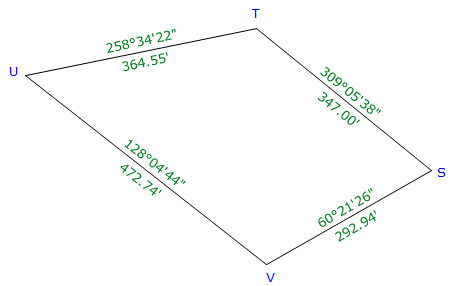Figure E-7 Azimuth Traverse Example

 Line Azimuth Length (ft) Lat (ft) Dep (ft) ST 309°05'38" 347.00 +218.816 -269.311 TU 258°34'22" 364.55 -72.226 -357.324 UV 128°04'44" 472.74 -291.560 +372.123 VS 60°21'26" 292.94 +144.885 +254.602 sums: 1477.23 -0.085 +0.090 Distance Lat err too far S Dep err too far E

(1) Adjust the Lats and Deps

Setup Equations E-1 and E-2:Solve Equations E-3 and E-4 for each line:

Line ST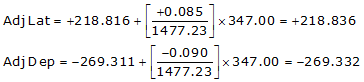Line TULine UV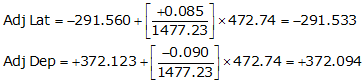Line VS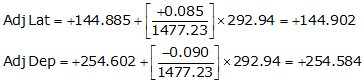Check the closure condition

 Adjusted Line Lat (ft) Dep (ft) ST +218.836 -269.332 TU -72.205 -357.346 UV -291.533 +372.094 VS +144.902 +254.584 sums: 0.000 0.000 check check

(2) Compute adjusted lengths and directions

Use Equations E-5 and E-6 along with Figure E-5 to compute the new length and direction for each line.

Line ST

Adj Lat = +218.836 <- North
Adj Dep = -269.332 <- WestBecause it's in the NW quadrant: Az = 360°00'00"+(-50°54'20.4") =309°05'39.6"

Line TU

Adj Lat = -72.205 <- South
Adj Dep = -357.346 <- West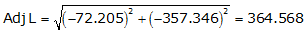Because it's in the SW quadrant: Az = 180°00'00"+(78°34'36.0") = 258°34'36.0"

Line UV

Adj Lat = -291.533 <- South
Adj Dep = +372.094 <- East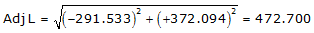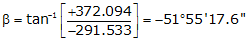Because it's in the SE quadrant: Az = 180°00'00"+(-51°55'17.6") = 128°04'42.4"

Line VS

Adj Lat = +144.902 <- North
Adj Dep = +254.584 <- East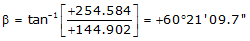Because it's in the NE quadrant: Az = 60°21'09.7"

 Adjusted Adjusted Line Lat (ft) Dep (ft) Length (ft) Azimuth ST +218.836 -269.332 347.029 309°05'39.6" TU -72.205 -357.346 364.568 258°34'36.0" UV -291.533 +372.094 472.700 128°04'42.4" VS +144.902 +254.584 292.933 60°21'09.7"

c. Crossing Loop Traverse

As long as a traverse closes back on its beginning point, it can be adjusted the same as any other loop traverse.Figure E-8 Crossing Loop Traverse Example

 Line Azimuth Length (ft) Lat (ft) Dep (ft) EF 133°02'45" 455.30 -310.780 +332.737 FG 24°33'35" 228.35 +207.691 +94.912 GH 241°05'15" 422.78 -204.403 -370.084 HE 349°25'20" 312.85 +307.534 -57.430 sums: 1419.28 +0.042 +0.135 Dist Lat err too far N Dep err too far E

(1) Adjust and recompute each line.

Setup Equations E-1 and E-2: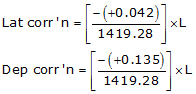Solve Equations E-3 and E-4 for each line:

Line EF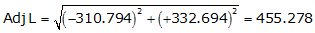Because it's in the SE quadrant: Az = 180°00'00"+(-46°56'57.1") = 133°03'02.9"

Line FG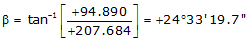Because it's in the NE quadrant: Az = 24°33'19.7"

Line GH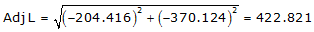Because it's in the SW quadrant: Az = 180°00'00"+(61°05'18.8") =241°05'18.8"

Line HE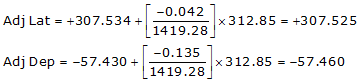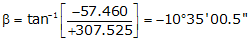Because it's in the NW quadrant: Az = 360°00'00"+(-10°35'00.5") = 349°24'59.5"